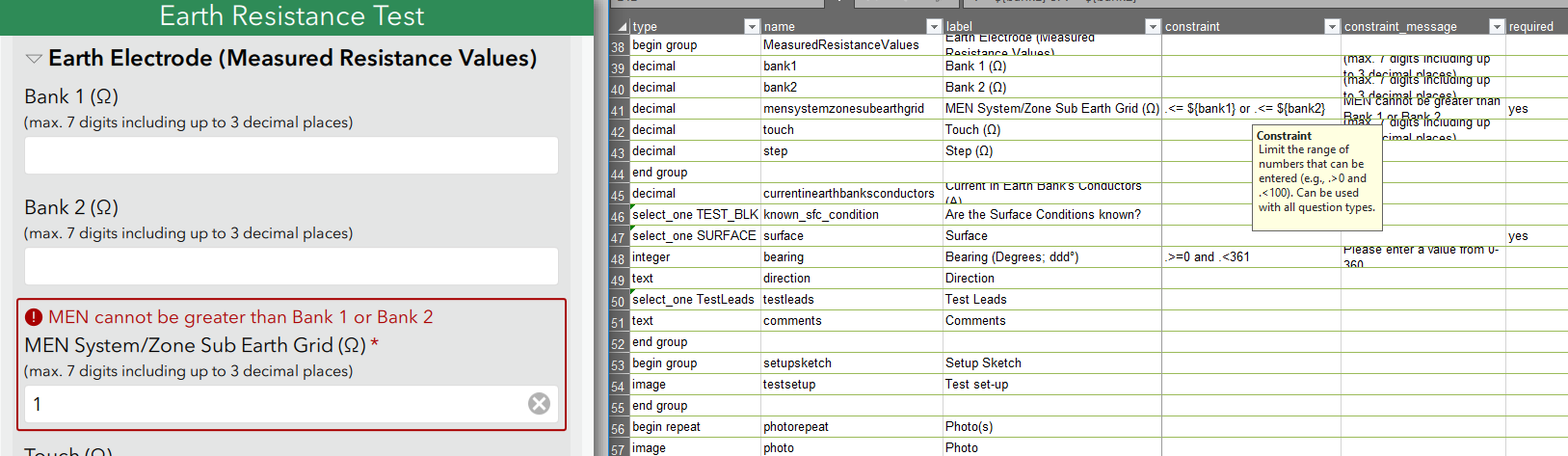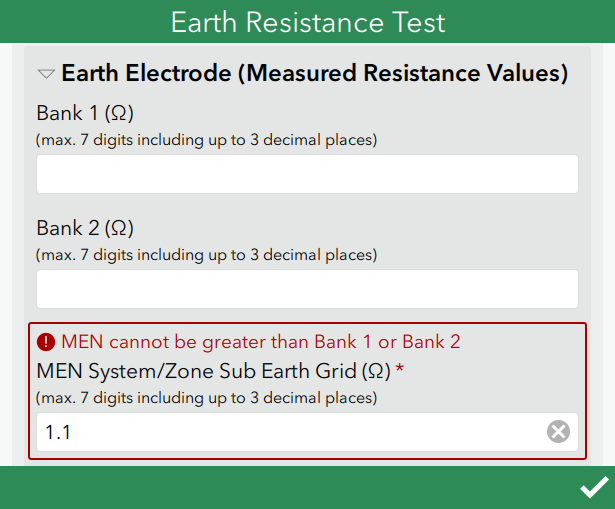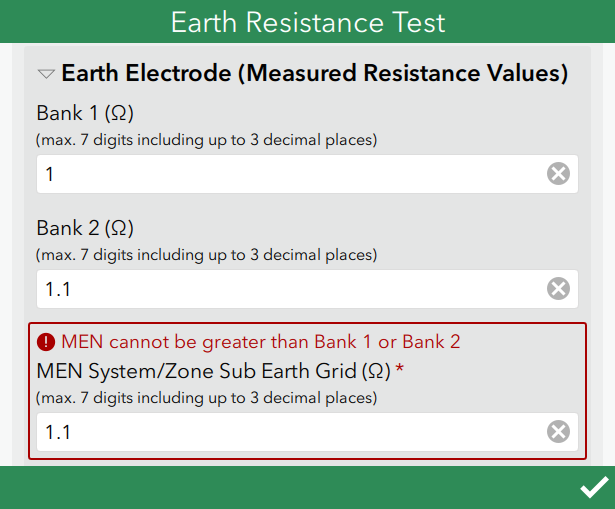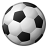# Can I make a conditional constraint?

4502
8
07-23-2019 04:10 PMOccasional Contributor

Can I make a conditional constraint?

I have a required field to record M.E.N. Resistance in Ohms.

I also have another two optional fields for Bank1 resistance and Bank2 resistance (Ohms).

Condition is M.E.N. cannot be greater than Bank1 or Bank2.

When Bank1 and/or Bank2 is empty, entering any value in M.E.N. will obviously be greater than Bank1 and/or Bank2.

Can I put an IF statement in the Constraint column?1 Solution

Accepted Solutionsby Anonymous User
Not applicable

Hi Roger,

I didn't cover all the cases you were expecting, it was intended to be an example expression so you could see how nested if statements work and try to adjust it yourself to get the result you were after.

You need to add an extra if statement at the start to check when both values are null, and ensure the value is 0 or greater for MEN, as you made this question required I assume it should always be a positive number. If not, and you expect negative numbers also, you may need to adjust this to work with any number. I have added this logic to the expression and tested it with Connect and App and it works:

if(string-length(\${bank1})=0 and string-length(\${bank2})=0

,.>=0
,if(string-length(\${bank1})>0 and string-length(\${bank2})>0
,.<=\${bank1} and .<=\${bank2}
,if(string-length(\${bank1})>0 and string-length(\${bank2})=0
,.<=\${bank1}
,if(string-length(\${bank2})>0 and string-length(\${bank1})=0
,.<=\${bank2}
,''
)
)
)
)

As for your second screenshot above, this does not make sense when compared with the message you display. The constraint message says that:

MEN cannot be greater than Bank 1 or Bank 2

In your example, Blank1 is "1", Blank2 is "1.1" and the MEN value is "1.1". This will cause the constraint to be true, as 1.1 is greater than 1. This occurs because we used an "and" statement in the expression were both Blank1 and Blank2 had values. If you want it to be an either or constraint, you then need to use an "or" statement. However for me, the constraint message is not clear in this case, as the way it reads would mean that MEN should not be greater than both the values, not just one or the other. In that case use the following expression with an "or" in the second if statement:

if(string-length(\${bank1})=0 and string-length(\${bank2})=0
,.>=0
,if(string-length(\${bank1})>0 and string-length(\${bank2})>0
,.<=\${bank1} or .<=\${bank2}
,if(string-length(\${bank1})>0 and string-length(\${bank2})=0
,.<=\${bank1}
,if(string-length(\${bank2})>0 and string-length(\${bank1})=0
,.<=\${bank2}
,''
)
)
)
)

Another way you could do the constraint checks would be to put the constraint messages on the Blank1 and Blank2 fields, that will simply things as you are not trying to validate two different values in the one field where either could be true and accepted.

Test out both the above examples, and you may need to adjust them, test for all cases of values, null, higher and lower values to ensure it works the way you are expecting.

Regards,

Phil.

8 Repliesby Anonymous User
Not applicable

Hi Roger,

Yes, you can use expressions (including if statements) in the constraint column. The same expressions that can be used in calculation column, required and read only columns, can also be used for constraints.

This means you can make your constraint conditional based on the answer to other questions in your survey.

Phil.Occasional Contributor

Thanks, Phil.

I'm showing my lack of programming skills, then, by asking how I would write an expression. So far I have come up with:

if(\${bank1} != NaN, .<= \${bank1}, if(\${bank2} != NaN, .<= \${bank2}, ''))

Which means: if bank1 is not 'not a number' then constrain to less than or equal to bank1, if bank2 is not 'not a number' then constrain to less than or equal to bank2, else do not constrain.

bank1 and bank2 are decimal fields, as is the M.E.N. field.

Am I on the right track? Probably not, because I get this:Because both bank1 and bank2 are NaN, any value in MEN should be acceptable. A condition should only be applied if either bank1 or bank2 have a number.

Thanks,

Roger.by Anonymous User
Not applicable

Hi Roger,

I finally had a quick look at your survey. Because you are wanting to use an "or" statement for blank1 or blank2, you can not use a nested if statement in the way you have constructed it, as once the first condition if false, it will always do nothing and skip the second condition. Therefore you need to use a nested if statement with an "and" that covers all the combinations of inputs in blank1 "and" blank2 plus I suggest you use string-length() instead of a NaN check. I tested the following in your survey and this works:

if(string-length(\${bank1})>0 and string-length(\${bank2})>0
,.<=\${bank1} and .<=\${bank2}
, if(string-length(\${bank1})>0 and string-length(\${bank2})=0
,.<=\${bank1}
, if(string-length(\${bank2})>0 and string-length(\${bank1})=0
,.<=\${bank2}
,''
)
)
)

If you want to put all on one line in your xlsx file it will look like:

if(string-length(\${bank1})>0 and string-length(\${bank2})>0,.<=\${bank1} and .<=\${bank2}, if(string-length(\${bank1})>0 and string-length(\${bank2})=0,.<=\${bank1}, if(string-length(\${bank2})>0 and string-length(\${bank1})=0,.<=\${bank2},'')))

This will cover the case where the there is null values in either blank1 or blank2 or both are null.

Hope this helps.

Phil.Occasional Contributor

Hi Phil,

I tried it and it hasn't quite worked. The constraint is still applied when both bank1 and bank2 are empty.The above should be OK.The above should also be OK because MEN is no higher than bank2.

Regards,

Rogerby Anonymous User
Not applicable

Hi Roger,

I didn't cover all the cases you were expecting, it was intended to be an example expression so you could see how nested if statements work and try to adjust it yourself to get the result you were after.

You need to add an extra if statement at the start to check when both values are null, and ensure the value is 0 or greater for MEN, as you made this question required I assume it should always be a positive number. If not, and you expect negative numbers also, you may need to adjust this to work with any number. I have added this logic to the expression and tested it with Connect and App and it works:

if(string-length(\${bank1})=0 and string-length(\${bank2})=0

,.>=0
,if(string-length(\${bank1})>0 and string-length(\${bank2})>0
,.<=\${bank1} and .<=\${bank2}
,if(string-length(\${bank1})>0 and string-length(\${bank2})=0
,.<=\${bank1}
,if(string-length(\${bank2})>0 and string-length(\${bank1})=0
,.<=\${bank2}
,''
)
)
)
)

As for your second screenshot above, this does not make sense when compared with the message you display. The constraint message says that:

MEN cannot be greater than Bank 1 or Bank 2

In your example, Blank1 is "1", Blank2 is "1.1" and the MEN value is "1.1". This will cause the constraint to be true, as 1.1 is greater than 1. This occurs because we used an "and" statement in the expression were both Blank1 and Blank2 had values. If you want it to be an either or constraint, you then need to use an "or" statement. However for me, the constraint message is not clear in this case, as the way it reads would mean that MEN should not be greater than both the values, not just one or the other. In that case use the following expression with an "or" in the second if statement:

if(string-length(\${bank1})=0 and string-length(\${bank2})=0
,.>=0
,if(string-length(\${bank1})>0 and string-length(\${bank2})>0
,.<=\${bank1} or .<=\${bank2}
,if(string-length(\${bank1})>0 and string-length(\${bank2})=0
,.<=\${bank1}
,if(string-length(\${bank2})>0 and string-length(\${bank1})=0
,.<=\${bank2}
,''
)
)
)
)

Another way you could do the constraint checks would be to put the constraint messages on the Blank1 and Blank2 fields, that will simply things as you are not trying to validate two different values in the one field where either could be true and accepted.

Test out both the above examples, and you may need to adjust them, test for all cases of values, null, higher and lower values to ensure it works the way you are expecting.

Regards,

Phil.Occasional Contributor

Hi Phil,

Thank you for your help, it is greatly appreciated. The second expression you created worked with the 'or' in the second if statement. It does highlight the clarity needed in question labels, hints, messages and notes!

Thanks and regards,

Rogerby Anonymous User
Not applicable

Great, glad it worked for you.Occasional Contributor III

This has been a huge help!  I was just wondering if it is possible to extend this into the constraint message?  I basically have a binary condition for the constraint, if "true" constraint x and y apply, if "false" only constraint x applies. I tried to create a couple text columns one with a constraint message for the true condition another for false and third calculated message to create the display constraint message fort the selected condition. e.g. if(\${postDate} = 'T', \${trueConstraintMsg}, \${falseConstraintMsg}) and then entered \${displayConstrainMsg} in the constrain_message column. Needless to say this didn't work, was wondering if there is another solution? Is the constraint_message column only able to take text and not a result from another question? If I can't make it happen through a constraint message I can just do it through a conditional note but it won't be a elegant.